# Daily Learning 01.07.2020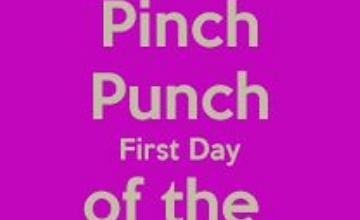Good morning!  Wow - we finally made it to July!  Let's make this last month of the academic year a really positive one!  What are your aims this month?

Here is a shoutout to Thay for his work yesterday.  Well done to everyone who is continuing to produce excellent work, like Thay.  CHALLENGE: How many adverbials can you spot in Thay's writing?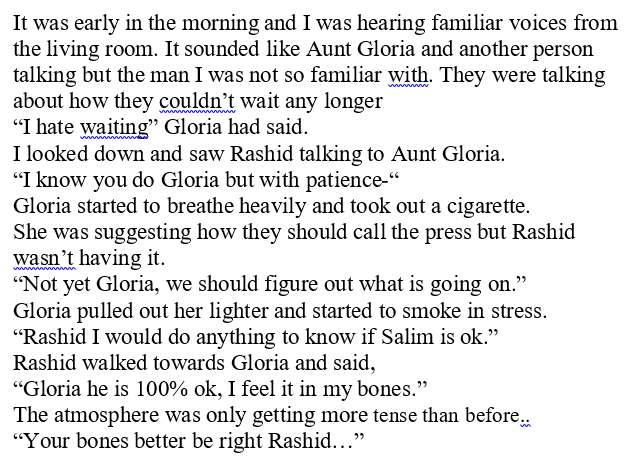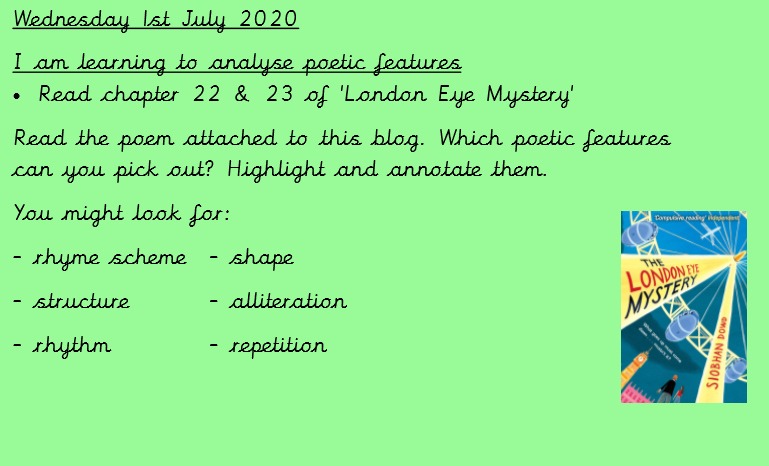After reading chapters 22 and 23, here is your English for today: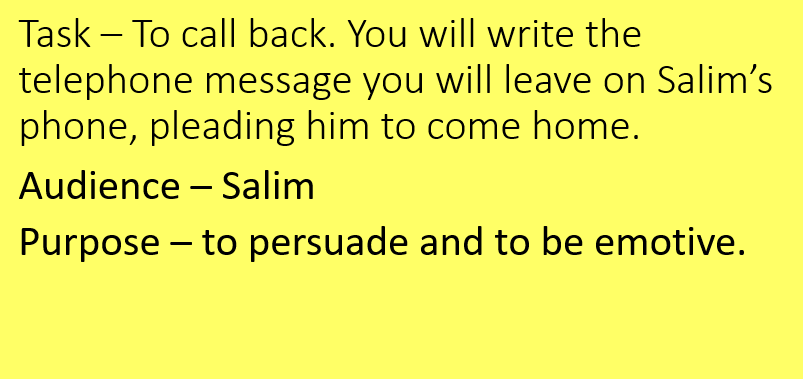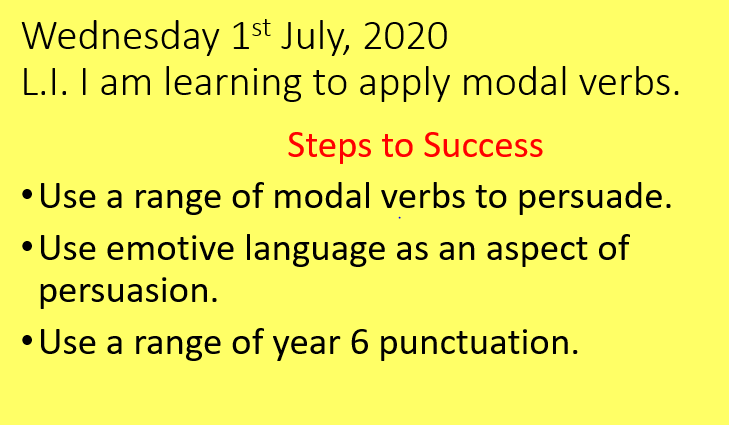Note: Year 6 punctuation includes colons, semi-colons, parenthesis (brackets, dashes or commas), hyphens and ellipses as well as your basic full stops, exclamation marks, capital letters, question marks and apostrophes correctly.  Challenge yourself!

Here is your Maths for today: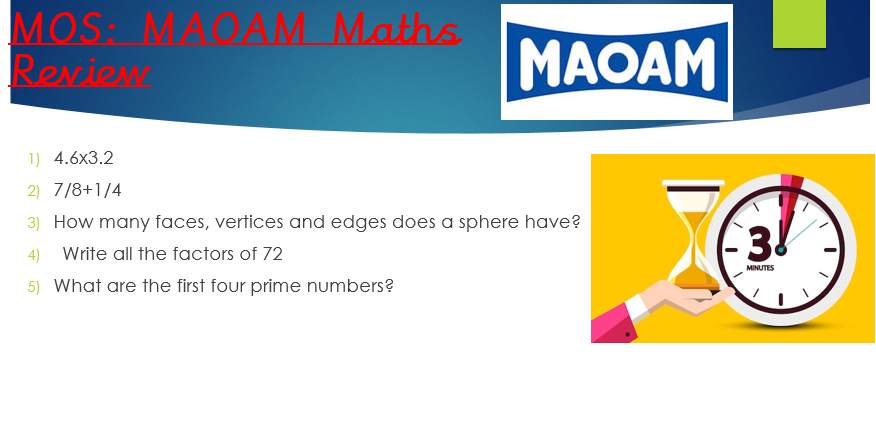Let's review the final 5 questions from Monday's Maoam Maths.

1) Multiplying decimals can be treated the same as multiplying by integers (whole numbers) if we multiply each decimal by 10 first.  Instead of 4.6 x 3.2 we can multiply each number by 10 to become 46 x 32 and then use the column method as we would normally (as long as we remember to make them 100 times smaller at the end; Why 100 and not 10?  Well, we've made the numbers 10 times bigger and then ten times bigger again, or a hundred times bigger in total). 46 x 32 = 1472 so 4.6 x 3.2 = 14.72

2) To add fractions, we need to make sure the denominators (bottom numbers) are the same.  In this case, they are not so we need to find equivalent fractions first.  1/4 is the same as 2/8 if both the numerator (top number) and denominator are doubled.  For 7/8 2/8, just add the numerators and keep the denominators: 9/8 (1 1/8)

3) A sphere has one face, no vertices and no edges... what a strange shape!

4)  Freddie Factor is perfect here!  72 has 12 factors: 1 x 72; 2 x 36; 3 x 24; 4 x 18; 6 x 12; 8 x 9

5) A prime number is an integer that has only 2 factors: 1 and itself.  The first four prime numbers are 2, 3, 5 and 7.  2 is the only even prime number as all the others are divisible by 2